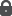A polynomial time primal network simplex algorithm for minimum cost flows
Orlin, James B.

 Data: 1997 Resum: Developing a polynomial time primal network simplex algorithm for the minimum cost flow problem has been a long standing open problem. In this paper, we develop one such algorithm that runs in O(min(n^2m log nC, n^2m^2 log n)) time, where n is the number of nodes in the network, m is the number of arcs, and C denotes the maximum absolute arc costs if arc costs are integer and (infin) otherwise. We first introduce a pseudopolynomial variant of the network simplex algorithm called the "premultiplier algorithm". We then develop a cost-scaling version of the premultiplier algorithm that solves the minimum cost flow problem in O(min(nm log nC, nm^2 log n)) pivots. With certain simple data structures, the average time per pivot can be shown to be O(n). We also show that the diameter of the network polytope is O(nm log n). . Drets: Tots els drets reservats.Llengua: Anglès Document: Article ; recerca ; Versió publicada Matèria: Minimum cost flows ; Network simplex ; Polynomial time ; Simplex algorithm ; Premultipliers Publicat a: Mathematical Programming, vol. 78 n. 2 (1997) p. 109-129, ISSN 0025-5610

 21 p, 1.1 MBAccés restringit a la UAB

El registre apareix a les col·leccions:
Articles > Articles de recerca
Articles > Articles publicats

Registre creat el 2006-03-13, darrera modificació el 2023-06-03

 #bookmark_sciencewise, #bookmark { float: left; } #bookmark_sciencewise li { padding: 2px; width: 25px} #bookmark_sciencewise ul, #bookmark ul { list-style-image: none; } @import "https://ddd.uab.cat/css/jquery.bookmark.css";Afegeix-lo al cistell personal Anomena i desa Citation, BibTeX, MARC, MARCXML, DC, EDM OpenAire4 #bookmark_sciencewise, #bookmark { float: left; } #bookmark_sciencewise li { padding: 2px; width: 25px} #bookmark_sciencewise ul, #bookmark ul { list-style-image: none; } @import "https://ddd.uab.cat/css/jquery.bookmark.css";